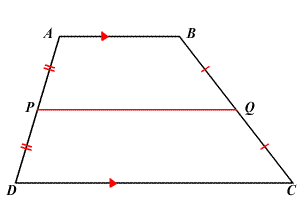# Midsegment of a Trapezoid

The midsegment of a trapezoid is the segment connecting the midpoints of the two non-parallel sides.

In trapezoid $ABCD$ below, segment $PQ$ is the midsegment.The length of the midsegment of trapezoid is half the sum of the lengths of the two parallel sides. In the figure above:

$PQ=\frac{AB+CD}{2}$